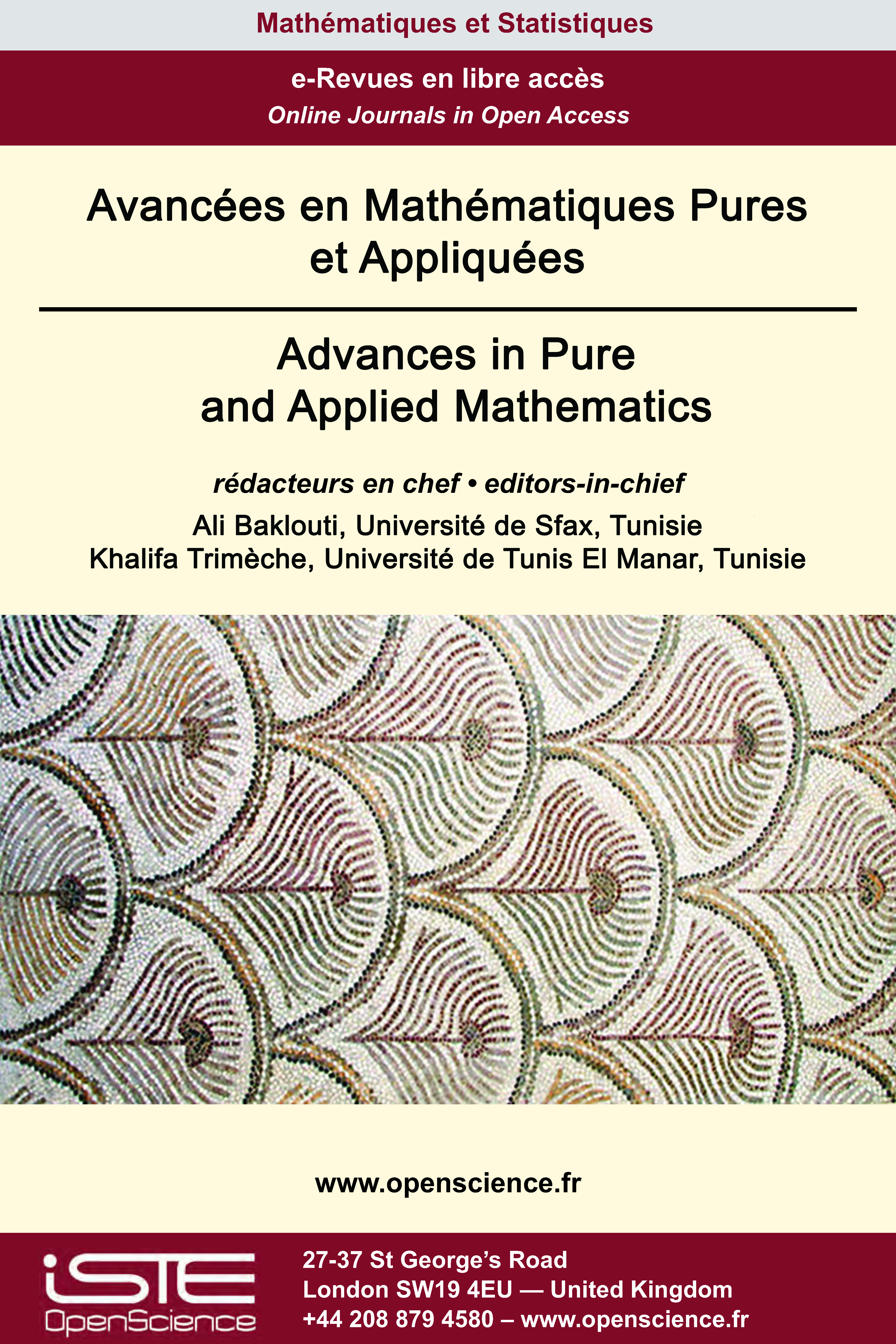# Vol 11 - Issue 2 (September 2020)

## List of Articles

Hereditary classes of ordered binary structures

Balogh, Bollobás and Morris (2006) have described a threshold phenomenon in the behavior of the profile of hereditary classes of ordered graphs. In this paper, we give an other look at their result based on the notion of monomorphic decomposition of a relational structure introduced in . We prove that the class 𝔖 of ordered binary structures which do not have a finite monomorphic decomposition has a finite basis (a subset 𝔄 such that every member of 𝔖 embeds some member of 𝔄). In the case of ordered reflexive directed graphs, the basis has 1242 members and the profile of their ages grows at least as the Fibonacci function. From this result, we deduce that the following dichotomy property holds for every hereditary class ℭ of finite ordered binary structures of a given finite type. Either there is an integer ℓ such that every member of ℭ has a monomorphic decomposition into at most ℓ blocks and in this case the profile of ℭ is bounded by a polynomial of degree ℓ − 1 (and in fact is a polynomial), or ℭ contains the age of a structure which does not have a finite monomorphic decomposition, in which case the profile of ℭ is bounded below by the Fibonacci function.

On some nonlocal elliptic systems with multiple parameters

In this paper, using sub-supersolution argument, we prove some existence results on positive solution for a class of nonlocal elliptic systems with multiple parameters in bounded domains.

Sharp bounds for Steklov eigenvalues on star-shaped domains

In this article, we consider Steklov eigenvalue problem on star-shaped bounded domain Ω in hypersurface of revolution and paraboloid, P = {(x, y, z) ∈ ℝ3 : z = x2 + y2}. A sharp lower bound is derived for all Steklov eigenvalues of Ω in terms of the Steklov eigenvalues of the largest geodesic ball contained in Ω with the same center as Ω. This work is a generalization of a result given by Kuttler and Sigillito (SIAM Rev 10:368 − 370, 1968) on a star-shaped bounded domain in ℝ2.

Global Small data Solutions for a system of semilinear heat equations and the corresponding system of damped wave equations with nonlinear memory

We consider the Cauchy problem for a strongly coupled semi-linear heat equations with some kind of nonlinearity in multi-dimensional space ℝN. We see under some conditions on the exponents and on the dimension N, that the existence and uniqueness of time-global solutions for small data and their asymptotic behaviors are obtained. This observation will be applied to the corresponding system of the damped wave equations in low dimensional space.### Other issues :

2020

Volume 20- 11

Issue 1 (May 2020)
Issue 2 (September 2020)

Forthcoming papers

2021

Volume 21- 12

Issue 1 (January 2021)
Issue 2 (May 2021)